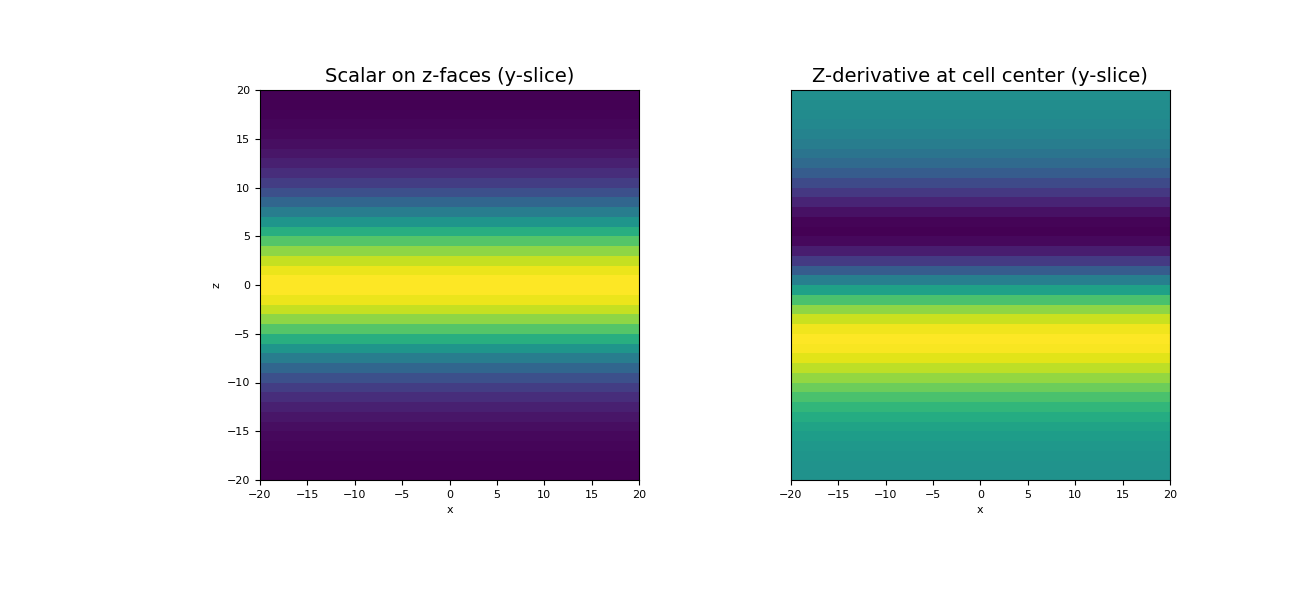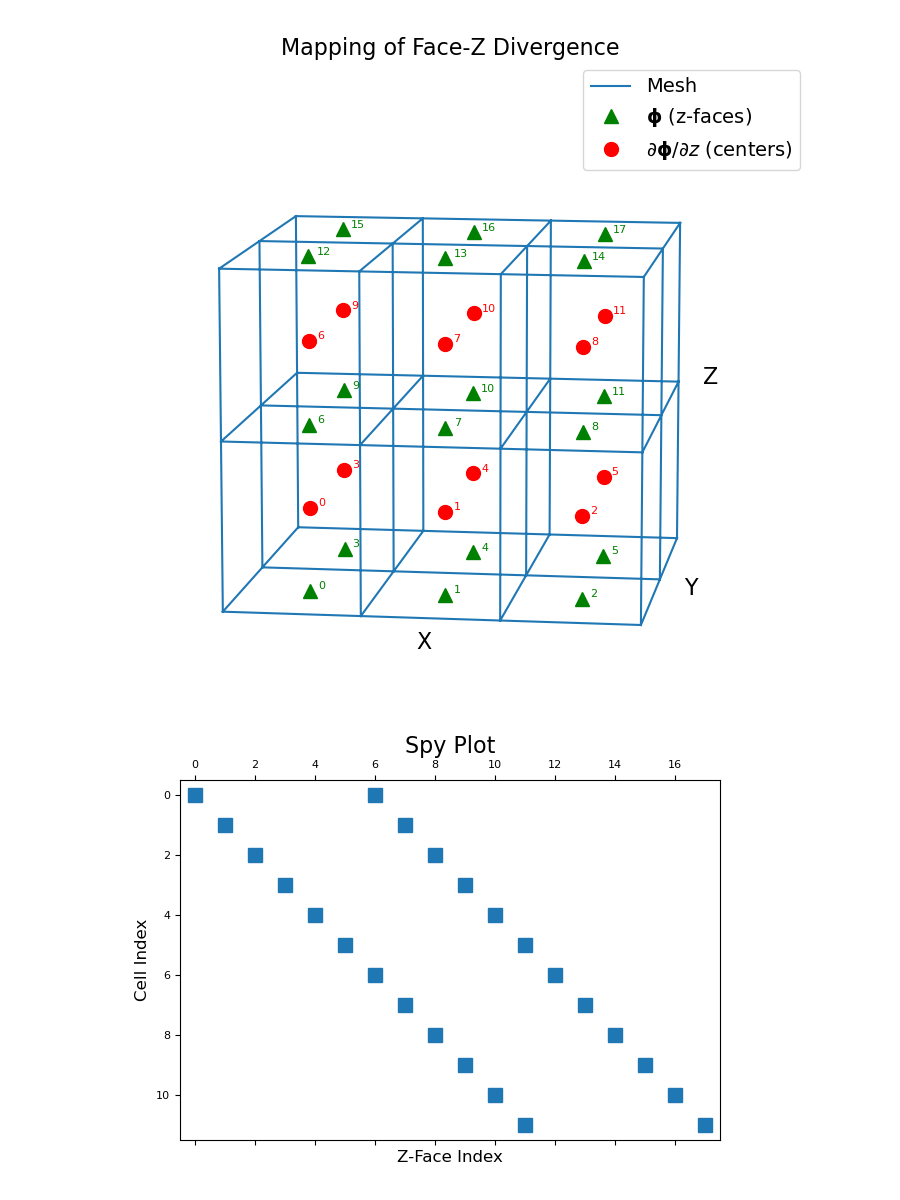# discretize.TensorMesh.face_z_divergence¶

property TensorMesh.face_z_divergence

Z-derivative operator (z-faces to cell-centres)

This property constructs a 2nd order z-derivative operator which maps from z-faces to cell centers. The operator is a sparse matrix $$\mathbf{D_z}$$ that can be applied as a matrix-vector product to a discrete scalar quantity $$\boldsymbol{\phi}$$ that lives on z-faces; i.e.:

dphi_dz = Dz @ phi


For a discrete vector whose z-component lives on z-faces, this operator can also be used to compute the contribution of the z-component toward the divergence.

Returns
(n_cells, n_faces_z) scipy.sparse.csr_matrix

The numerical z-derivative operator from z-faces to cell centers

Examples

Below, we demonstrate 2) how to apply the face-z divergence operator, and 2) the mapping of the face-z divergence operator and its sparsity. Our example is carried out on a 3D mesh.

We start by importing the necessary packages and modules.

>>> from discretize import TensorMesh
>>> import numpy as np
>>> import matplotlib.pyplot as plt
>>> import matplotlib as mpl


For a discrete scalar quantity $$\boldsymbol{\phi}$$ defined on the z-faces, we take the z-derivative by constructing the face-z divergence operator and multiplying as a matrix-vector product.

>>> h = np.ones(40)
>>> mesh = TensorMesh([h, h, h], "CCC")


Create a discrete quantity on z-faces

>>> faces_z = mesh.faces_z
>>> phi = np.exp(-(faces_z[:, 2] ** 2) / 8** 2)


Construct the z-divergence operator and apply to vector

>>> Dz = mesh.face_z_divergence
>>> dphi_dz = Dz @ phi


Plot the original function and the z-divergence

Expand to show scripting for plot
>>> fig = plt.figure(figsize=(13, 6))
>>> w = np.r_[np.ones(mesh.nFx+mesh.nFz), phi]  # Need vector on all faces for image plot
>>> mesh.plot_slice(w, ax=ax1, v_type="Fz", normal='Y', ind=20)
>>> ax1.set_title("Scalar on z-faces (y-slice)", fontsize=14)
>>> mesh.plot_slice(dphi_dz, ax=ax2, normal='Y', ind=20)
>>> ax2.set_yticks([])
>>> ax2.set_ylabel("")
>>> ax2.set_title("Z-derivative at cell center (y-slice)", fontsize=14)
>>> plt.show()The discrete z-face divergence operator is a sparse matrix that maps from z-faces to cell centers. To demonstrate this, we construct a small 3D mesh. We then show the ordering of the elements in the original discrete quantity $$\boldsymbol{\phi}$$ and its z-derivative $$\partial \boldsymbol{\phi}/ \partial z$$ as well as a spy plot.

Expand to show scripting for plot
>>> mesh = TensorMesh([[(1, 3)], [(1, 2)], [(1, 2)]])
>>> fig = plt.figure(figsize=(9, 12))
>>> ax1 = fig.add_axes([0, 0.35, 1, 0.6], projection='3d', elev=10, azim=-82)
>>> mesh.plot_grid(ax=ax1)
>>> ax1.plot(
...     mesh.faces_z[:, 0], mesh.faces_z[:, 1], mesh.faces_z[:, 2], "g^", markersize=10
... )
>>> for ii, loc in zip(range(mesh.nFz), mesh.faces_z):
...     ax1.text(loc + 0.05, loc + 0.05, loc, "{0:d}".format(ii), color="g")

>>> ax1.plot(
...    mesh.cell_centers[:, 0], mesh.cell_centers[:, 1], mesh.cell_centers[:, 2],
...    "ro", markersize=10
... )
>>> for ii, loc in zip(range(mesh.nC), mesh.cell_centers):
...     ax1.text(loc + 0.05, loc + 0.05, loc, "{0:d}".format(ii), color="r")
>>> ax1.legend(
...     ['Mesh','$\mathbf{\phi}$ (z-faces)','$\partial \mathbf{\phi}/\partial z$ (centers)'],
...     loc='upper right', fontsize=14
... )


Manually make axis properties invisible

>>> ax1.set_xticks([])
>>> ax1.set_yticks([])
>>> ax1.set_zticks([])
>>> ax1.w_xaxis.set_pane_color((1.0, 1.0, 1.0, 0.0))
>>> ax1.w_yaxis.set_pane_color((1.0, 1.0, 1.0, 0.0))
>>> ax1.w_zaxis.set_pane_color((1.0, 1.0, 1.0, 0.0))
>>> ax1.w_xaxis.line.set_color((1.0, 1.0, 1.0, 0.0))
>>> ax1.w_yaxis.line.set_color((1.0, 1.0, 1.0, 0.0))
>>> ax1.w_zaxis.line.set_color((1.0, 1.0, 1.0, 0.0))
>>> ax1.set_title("Mapping of Face-Z Divergence", fontsize=16, pad=-15)


Spy plot the operator,

>>> ax2 = fig.add_axes([0.05, 0.05, 0.9, 0.3])
>>> ax2.spy(mesh.face_z_divergence)